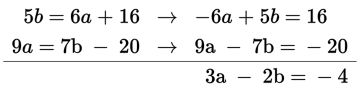# SAT Math Multiple Choice Question 798: Answer and Explanation

### Test Information

Question: 798

3. If 5b = 6a + 16 and 9a = 7b – 20, then what is the value of 3a – 2b?

• A. –8
• B. –4
• C. 4
• D. 8

Explanation:

B

Difficulty: Easy

Category: Heart of Algebra / Systems of Linear Equations

Strategic Advice: Whenever you're asked for an unusual quantity, rather than just the value of one variable or the other, there's a good chance that a time-saving shortcut (like simply adding or subtracting the equations) exists.

Getting to the Answer: Start by rewriting the equations so that they are in the same form. After doing so, if you look closely at the coefficients, you'll see that adding the two equations will yield exactly what you're asked to find:The sum of the two equations tells you that 3a - 2b is -4, making (B) the correct answer.